Filter
(found 10567 products)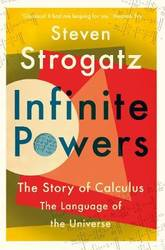Shortlisted for the Royal Society Science Book Prize 2019 A magisterial history of calculus (and the people behind it) from one of the world's foremost mathematicians. This is the captivating story of mathematics' greatest ever idea: calculus. Without it, there would be no computers, no microwave ovens, no GPS, and ...
Infinite Powers: The Story of Calculus - The Language of the Universe
Shortlisted for the Royal Society Science Book Prize 2019 A magisterial history of calculus (and the people behind it) from one of the world's foremost mathematicians. This is the captivating story of mathematics' greatest ever idea: calculus. Without it, there would be no computers, no microwave ovens, no GPS, and no space travel. But before it gave modern man almost infinite powers, calculus was behind centuries of controversy, competition, and even death. Taking us on a thrilling journey through three millennia, professor Steven Strogatz charts the development of this seminal achievement from the days of Archimedes to today's breakthroughs in chaos theory and artificial intelligence. Filled with idiosyncratic characters from Pythagoras to Fourier, Infinite Powers is a compelling human drama that reveals the legacy of calculus on nearly every aspect of modern civilisation, including science, politics, medicine, philosophy, and much besides.
15.74 USD

## Infinite Powers: The Story of Calculus - The Language of the Universe

by Steven Strogatz
Paperback / softback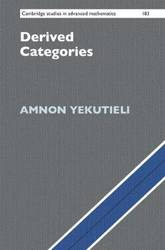There have been remarkably few systematic expositions of the theory of derived categories since its inception in the work of Grothendieck and Verdier in the 1960s. This book is the first in-depth treatment of this important component of homological algebra. It carefully explains the foundations in detail before moving on ...
Derived Categories
There have been remarkably few systematic expositions of the theory of derived categories since its inception in the work of Grothendieck and Verdier in the 1960s. This book is the first in-depth treatment of this important component of homological algebra. It carefully explains the foundations in detail before moving on to key applications in commutative and noncommutative algebra, many otherwise unavailable outside of research articles. These include commutative and noncommutative dualizing complexes, perfect DG modules, and tilting DG bimodules. Written with graduate students in mind, the emphasis here is on explicit constructions (with many examples and exercises) as opposed to axiomatics, with the goal of demystifying this difficult subject. Beyond serving as a thorough introduction for students, it will serve as an important reference for researchers in algebra, geometry and mathematical physics.
89.240000 USD

## Derived Categories

by Amnon Yekutieli
Hardback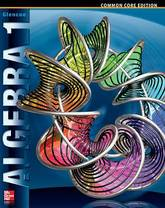The only program that supports the Common Core State Standards throughout four-years of high school mathematics with an unmatched depth of resources and adaptive technology that helps you differentiate instruction for every student. Connects students to math content with print, digital and interactive resources. Prepares students to meet the rigorous ...
Algebra 1, Student Edition
The only program that supports the Common Core State Standards throughout four-years of high school mathematics with an unmatched depth of resources and adaptive technology that helps you differentiate instruction for every student. Connects students to math content with print, digital and interactive resources. Prepares students to meet the rigorous Common Core Standards with aligned content and focus on Standards of Mathematical Practice. Meets the needs of every student with resources that enable you to tailor your instruction at the classroom and indivdual level. Assesses student mastery and achievement with dynamic, digital assessment and reporting. Includes Print Student Edition
183.750000 USD

## Algebra 1, Student Edition

by McGraw-Hill
HardbackThis book offers an introduction to the theory of groupoids and their representations encompassing the standard theory of groups. Using a categorical language, developed from simple examples, the theory of finite groupoids is shown to knit neatly with that of groups and their structure as well as that of their ...
An Introduction to Groups, Groupoids and Their Representations
This book offers an introduction to the theory of groupoids and their representations encompassing the standard theory of groups. Using a categorical language, developed from simple examples, the theory of finite groupoids is shown to knit neatly with that of groups and their structure as well as that of their representations is described. The book comprises numerous examples and applications, including well-known games and puzzles, databases and physics applications. Key concepts have been presented using only basic notions so that it can be used both by students and researchers interested in the subject. Category theory is the natural language that is being used to develop the theory of groupoids. However, categorical presentations of mathematical subjects tend to become highly abstract very fast and out of reach of many potential users. To avoid this, foundations of the theory, starting with simple examples, have been developed and used to study the structure of finite groups and groupoids. The appropriate language and notions from category theory have been developed for students of mathematics and theoretical physics. The book presents the theory on the same level as the ordinary and elementary theories of finite groups and their representations, and provides a unified picture of the same. The structure of the algebra of finite groupoids is analysed, along with the classical theory of characters of their representations. Unnecessary complications in the formal presentation of the subject are avoided. The book offers an introduction to the language of category theory in the concrete setting of finite sets. It also shows how this perspective provides a common ground for various problems and applications, ranging from combinatorics, the topology of graphs, structure of databases and quantum physics.
195.27 USD

## An Introduction to Groups, Groupoids and Their Representations

by Alberto Ibort
Hardback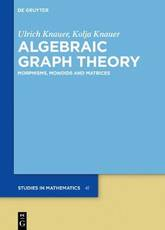Graph models are extremely useful for a large number of applications as they play an important role as structuring tools. They allow to model net structures - like roads, computers, telephones, social networks - instances of abstract data structures - like lists, stacks, trees - and functional or object oriented ...
Algebraic Graph Theory: Morphisms, Monoids and Matrices
Graph models are extremely useful for a large number of applications as they play an important role as structuring tools. They allow to model net structures - like roads, computers, telephones, social networks - instances of abstract data structures - like lists, stacks, trees - and functional or object oriented programming. The focus of this highly self-contained book is on homomorphisms and endomorphisms, matrices and eigenvalues.
125.990000 USD

## Algebraic Graph Theory: Morphisms, Monoids and Matrices

by Kolja Knauer, Ulrich Knauer
Hardback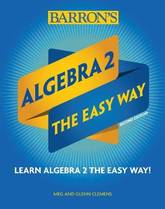This new edition in Barron's E-Z Series contains everything students need to succeed in an algebra 2 class. E-Z Algebra 2 covers the following topics: Linear Functions, Absolute Value Functions, Polynomial Operations, Quadratic Functions, Complex Numbers, Functions and Relations, Polynomial Functions, Radicals, Rational Functions, Exponential Functions, Logarithmic Functions, Series and ...
Algebra 2: The Easy Way
This new edition in Barron's E-Z Series contains everything students need to succeed in an algebra 2 class. E-Z Algebra 2 covers the following topics: Linear Functions, Absolute Value Functions, Polynomial Operations, Quadratic Functions, Complex Numbers, Functions and Relations, Polynomial Functions, Radicals, Rational Functions, Exponential Functions, Logarithmic Functions, Series and Sequences, and Statistics and Modeling. Review exercises are at the end of each chapter. The E-Z Series presents new, updated, and improved versions of Barron's longtime popular Easy Way books. Updated cover designs, interior layouts, and more graphic material than ever make these books ideal as self-teaching manuals. Teachers have discovered that E-Z titles also make excellent supplements to classroom textbooks. Skill levels range between senior high school and college-101 standards. All titles in the series present detailed reviews of the target subject plus short quizzes and longer tests to help students assess their learning progress.
17.840000 USD

## Algebra 2: The Easy Way

by Glenn Clemens, Meg Clemens
Paperback / softback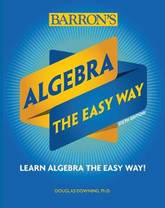This new edition in Barron's E-Z Series contains everything students need to prepare themselves for an algebra class. E-Z Algebra provides key content review and practice exercises to help students master algebra. Topics covered in this detailed review of algebra include general rules for dealing with numbers, equations, negative numbers ...
Algebra: The Easy Way
This new edition in Barron's E-Z Series contains everything students need to prepare themselves for an algebra class. E-Z Algebra provides key content review and practice exercises to help students master algebra. Topics covered in this detailed review of algebra include general rules for dealing with numbers, equations, negative numbers and integers, fractions and rational numbers, exponents, roots and real numbers, algebraic expressions, functions, graphs, systems of two equations, quadratic equations, circles, ellipses, parabolas, polynomials, numerical series, permutations, combinations, the binomial formula, proofs by mathematical induction, exponential functions and logarithms, simultaneous equations and matrices, and imaginary numbers. The E-Z Series presents new, updated, and improved versions of Barron's longtime popular Easy Way books. Updated cover designs, interior layouts, and more graphic material than ever make these books ideal as self-teaching manuals. Teachers have discovered that E-Z titles also make excellent supplements to classroom textbooks. Skill levels range between senior high school and college-101 standards. All titles in the series present detailed reviews of the target subject plus short quizzes and longer tests to help students assess their learning progress.
17.840000 USD

## Algebra: The Easy Way

by Douglas Downing
Paperback / softback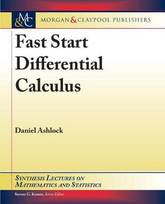This book reviews the algebraic prerequisites of calculus, including solving equations, lines, quadratics, functions, logarithms, and trig functions.It introduces the derivative using the limit-based definition and covers the standard function library and the product, quotient, and chain rules. It explores the applications of the derivative to curve sketching and optimization ...
Fast Start Differential Calculus
This book reviews the algebraic prerequisites of calculus, including solving equations, lines, quadratics, functions, logarithms, and trig functions.It introduces the derivative using the limit-based definition and covers the standard function library and the product, quotient, and chain rules. It explores the applications of the derivative to curve sketching and optimization and concludes with the formal definition of the limit, the squeeze theorem, and the mean value theorem.
41.950000 USD

## Fast Start Differential Calculus

by Daniel Ashlock
Paperback / softback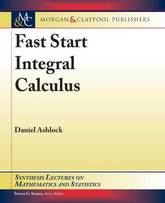This book introduces integrals, the fundamental theorem of calculus, initial value problems, and Riemann sums.It introduces properties of polynomials, including roots and multiplicity, and uses them as a framework for introducing additional calculus concepts including Newton's method, L'Hopital's Rule, and Rolle's theorem. Both the differential and integral calculus of parametric, ...
Fast Start Integral Calculus
This book introduces integrals, the fundamental theorem of calculus, initial value problems, and Riemann sums.It introduces properties of polynomials, including roots and multiplicity, and uses them as a framework for introducing additional calculus concepts including Newton's method, L'Hopital's Rule, and Rolle's theorem. Both the differential and integral calculus of parametric, polar, and vector functions are introduced. The book concludes with a survey of methods of integration, including u-substitution, integration by parts, special trigonometric integrals, trigonometric substitution, and partial fractions.
62.950000 USD

## Fast Start Integral Calculus

by Daniel Ashlock
HardbackThis book reviews the algebraic prerequisites of calculus, including solving equations, lines, quadratics, functions, logarithms, and trig functions.It introduces the derivative using the limit-based definition and covers the standard function library and the product, quotient, and chain rules. It explores the applications of the derivative to curve sketching and optimization ...
Fast Start Differential Calculus
This book reviews the algebraic prerequisites of calculus, including solving equations, lines, quadratics, functions, logarithms, and trig functions.It introduces the derivative using the limit-based definition and covers the standard function library and the product, quotient, and chain rules. It explores the applications of the derivative to curve sketching and optimization and concludes with the formal definition of the limit, the squeeze theorem, and the mean value theorem.
62.950000 USD

## Fast Start Differential Calculus

by Daniel Ashlock
Hardback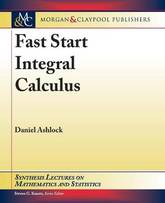This book introduces integrals, the fundamental theorem of calculus, initial value problems, and Riemann sums.It introduces properties of polynomials, including roots and multiplicity, and uses them as a framework for introducing additional calculus concepts including Newton's method, L'Hopital's Rule, and Rolle's theorem. Both the differential and integral calculus of parametric, ...
Fast Start Integral Calculus
This book introduces integrals, the fundamental theorem of calculus, initial value problems, and Riemann sums.It introduces properties of polynomials, including roots and multiplicity, and uses them as a framework for introducing additional calculus concepts including Newton's method, L'Hopital's Rule, and Rolle's theorem. Both the differential and integral calculus of parametric, polar, and vector functions are introduced. The book concludes with a survey of methods of integration, including u-substitution, integration by parts, special trigonometric integrals, trigonometric substitution, and partial fractions.
41.950000 USD

## Fast Start Integral Calculus

by Daniel Ashlock
Paperback / softback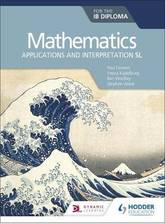Enable students to construct mathematical models by exploring challenging problems and the use of technology. - Engage and excite students with examples and photos of maths in the real world, plus inquisitive starter activities to encourage their problem-solving skills. - Build mathematical thinking with our 'Toolkit' and mathematical exploration chapter, ...
Mathematics for the IB Diploma: Applications and interpretation SL: Applications and interpretation SL
Enable students to construct mathematical models by exploring challenging problems and the use of technology. - Engage and excite students with examples and photos of maths in the real world, plus inquisitive starter activities to encourage their problem-solving skills. - Build mathematical thinking with our 'Toolkit' and mathematical exploration chapter, along with our new toolkit feature of questions, investigations and activities. - Develop understanding with key concepts and applications integrated throughout, along with TOK links for every topic. - Prepare your students for assessment with worked examples, extended essay support and colour-coded questions to highlight the level of difficulty and the different types of questions. - Check understanding with review exercise midway and at the end of the textbook. Follows the new 2019 IB Guide for Mathematics: applications and interpretation Standard Level Available in the series Mathematics for the IB Diploma: Analysis and approaches SL Student Book ISBN: 9781510462359 Student eTextbook ISBN: 9781510461895 Whiteboard eTextbook ISBN: 9781510461901 Mathematics for the IB Diploma: Analysis and approaches HL Student Book ISBN: 9781510462366 Student eTextbook ISBN: 9781510461857 Whiteboard eTextbook ISBN: 9781510461864 SL & HL Teaching & Learning Resources ISBN: 9781510461918 Mathematics for the IB Diploma: Applications and interpretation SL Student Book ISBN: 9781510462380 Student eTextbook ISBN: 9781510461994 Whiteboard eTextbook ISBN: 9781510462007 Mathematics for the IB Diploma: Applications and interpretation HL Student Book ISBN: 9781510462373 Student eTextbook ISBN: 9781510461956 Whiteboard eTextbook ISBN: 9781510461963 SL and HL Teaching & Learning Resources ISBN: 9781510462014 Dynamic learning packages (include Teaching & Learning resources and Whiteboard eTextbooks) Analysis & approaches SL & HL ISBN: 9781510461925 Applications and interpretation SL and HL ISBN: 9781510462021 Analysis & approaches SL & HL and Applications and interpretation SL and HL ISBN: 9781510468474
72.09 USD

## Mathematics for the IB Diploma: Applications and interpretation SL: Applications and interpretation SL

by Stephen Ward, Ben Woolley, Vesna Kadelburg, Paul Fannon
Paperback / softbackThis textbook explains the fundamental concepts and techniques of group theory by making use of language familiar to physicists. Calculation methods in the context of physics are emphasized. New materials drawn from the teaching and research experience of the author are included. The generalized Gel'fand's method is presented to calculate ...
Group Theory For Physicists
This textbook explains the fundamental concepts and techniques of group theory by making use of language familiar to physicists. Calculation methods in the context of physics are emphasized. New materials drawn from the teaching and research experience of the author are included. The generalized Gel'fand's method is presented to calculate the matrices of irreducible representations of the simple Lie algebra and its Clebsch-Gordan coefficients. This book is for graduate students and young researchers in physics, especially theoretical physics. It is also for graduate students in theoretical chemistry.
111.58 USD

## Group Theory For Physicists

by Zhong-Qi Ma
Paperback / softback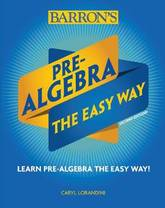This new edition in Barron's E-Z Series contains everything students need to prepare themselves for an algebra class. E-Z Pre-Algebra provides key content review, short quizzes, and practice tests to help students master pre-algebra. Separate chapters focus on fractions, integers, ratios, proportions, expressions, equations, inequalities, graphing, statistics and probability basics, ...
Pre-Algebra: The Easy Way
This new edition in Barron's E-Z Series contains everything students need to prepare themselves for an algebra class. E-Z Pre-Algebra provides key content review, short quizzes, and practice tests to help students master pre-algebra. Separate chapters focus on fractions, integers, ratios, proportions, expressions, equations, inequalities, graphing, statistics and probability basics, word problems, and more. Review questions and chapter reviews all have clear answers and explanations. The E-Z Series presents new, updated, and improved versions of Barron's longtime popular Easy Way books. Updated cover designs, interior layouts, and more graphic material than ever make these books ideal as self-teaching manuals. Teachers have discovered that E-Z titles also make excellent supplements to classroom textbooks. Skill levels range between senior high school and college-101 standards. All titles in the series present detailed reviews of the target subject plus short quizzes and longer tests to help students assess their learning progress.
17.840000 USD

## Pre-Algebra: The Easy Way

by Caryl Lorandini
Paperback / softback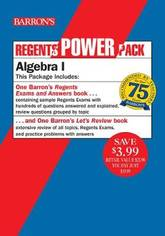Barron's Regents Algebra I Power Pack is the only Algebra I Regents study guide that gives students and teachers comprehensive content review and actual Regents exams as practice. This two-book set provides the key Algebra I content, step-by-step skills review, and real practice that students need to feel prepared for ...
Regents Algebra I Power Pack: Let's Review Algebra I + Regents Exams and Answers: Algebra I
Barron's Regents Algebra I Power Pack is the only Algebra I Regents study guide that gives students and teachers comprehensive content review and actual Regents exams as practice. This two-book set provides the key Algebra I content, step-by-step skills review, and real practice that students need to feel prepared for the test. Regents Exams and Answers: Algebra I includes: Six actual, administered Regents exams so students can get familiar with the test Comprehensive review questions grouped by topic, to help students refresh skills learned in class Thorough explanations for all answers Score analysis charts to help identify strengths and weaknesses Study tips and test-taking strategies Let's Review: Algebra I includes: Extensive review of all topics on the test Extra exercise problems with answers to help students refresh the skills they learned in class The Power Pack offers a savings of \$3.99 as compared to purchasing the books separately.
20.990000 USD

## Regents Algebra I Power Pack: Let's Review Algebra I + Regents Exams and Answers: Algebra I

by Gary M. Rubinstein
Paperback / softback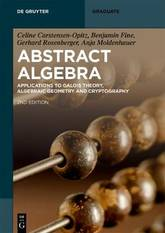A new approach to conveying abstract algebra, the area that studies algebraic structures, such as groups, rings, fields, modules, vector spaces, and algebras, that is essential to various scientific disciplines such as particle physics and cryptology. It provides a well written account of the theoretical foundations and it also includes ...
Abstract Algebra: Applications to Galois Theory, Algebraic Geometry, Representation Theory and Cryptography
A new approach to conveying abstract algebra, the area that studies algebraic structures, such as groups, rings, fields, modules, vector spaces, and algebras, that is essential to various scientific disciplines such as particle physics and cryptology. It provides a well written account of the theoretical foundations and it also includes a chapter on cryptography. End of chapter problems help readers with accessing the subjects.
60.890000 USD

## Abstract Algebra: Applications to Galois Theory, Algebraic Geometry, Representation Theory and Cryptography

by Gerhard Rosenberger, Anja Moldenhauer, Benjamin Fine, Celine Carstensen-Opitz
Paperback / softback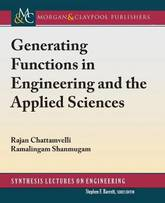This is an introductory book on generating functions (GFs) and their applications.It discusses commonly encountered generating functions in engineering and applied sciences, such as ordinary generating functions (OGF), exponential generating functions (EGF), probability generating functions (PGF), etc. Some new GFs like Pochhammer generating functions for both rising and falling factorials ...
Generating Functions in Engineering and the Applied Sciences
This is an introductory book on generating functions (GFs) and their applications.It discusses commonly encountered generating functions in engineering and applied sciences, such as ordinary generating functions (OGF), exponential generating functions (EGF), probability generating functions (PGF), etc. Some new GFs like Pochhammer generating functions for both rising and falling factorials are introduced in Chapter 2. Two novel GFs called mean deviation generating function (MDGF) and survival function generating function (SFGF), are introduced in Chapter 3. The mean deviation of a variety of discrete distributions are derived using the MDGF. The last chapter discusses a large number of applications in various disciplines including algebra, analysis of algorithms, polymer chemistry, combinatorics, graph theory, number theory, reliability, epidemiology, bio-informatics, genetics, management, economics, and statistics. Some background knowledge on GFs is often assumed for courses in analysis of algorithms, advanced data structures, digital signal processing (DSP), graph theory, etc. These are usually provided by either a course on discrete mathematics or introduction to combinatorics. But, GFs are also used in automata theory, bio-informatics, differential equations, DSP, number theory, physical chemistry, reliability engineering, stochastic processes, and so on. Students of these courses may not have exposure to discrete mathematics or combinatorics. This book is written in such a way that even those who do not have prior knowledge can easily follow through the chapters, and apply the lessons learned in their respective disciplines. The purpose is to give a broad exposure to commonly used techniques of combinatorial mathematics, highlighting applications in a variety of disciplines.
47.200000 USD

## Generating Functions in Engineering and the Applied Sciences

by Ramalingam Shanmugam, Rajan Chattamvelli
Paperback / softback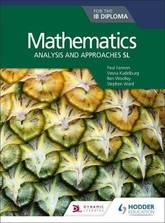Enable students to construct, communicate and justify correct mathematical arguments, with a range of activities and examples of maths in the real world. - Engage and excite students with examples and photos of maths in the real world, plus inquisitive starter activities to encourage their problem-solving skills - Build mathematical ...
Mathematics for the IB Diploma: Analysis and approaches SL: Analysis and approaches SL
Enable students to construct, communicate and justify correct mathematical arguments, with a range of activities and examples of maths in the real world. - Engage and excite students with examples and photos of maths in the real world, plus inquisitive starter activities to encourage their problem-solving skills - Build mathematical thinking with our 'Toolkit' and mathematical exploration chapter, along with our new toolkit feature of questions, investigations and activities - Develop understanding with key concepts and applications integrated throughout, along with TOK links for every topic - Prepare your students for assessment with worked examples, and extended essay support - Check understanding with review exercise midway and at the end of the coursebook Follows the new 2019 IB Guide for Mathematics: analysis and approaches Standard Level Available in the series Mathematics for the IB Diploma: Analysis and approaches SL Student Book ISBN: 9781510462359 Student eTextbook ISBN: 9781510461895 Whiteboard eTextbook ISBN: 9781510461901 Mathematics for the IB Diploma: Analysis and approaches HL Student Book ISBN: 9781510462366 Student eTextbook ISBN: 9781510461857 Whiteboard eTextbook ISBN: 9781510461864 SL & HL Teaching & Learning Resources ISBN: 9781510461918 Mathematics for the IB Diploma: Applications and interpretation SL Student Book ISBN: 9781510462380 Student eTextbook ISBN: 9781510461994 Whiteboard eTextbook ISBN: 9781510462007 Mathematics for the IB Diploma: Applications and interpretation HL Student Book ISBN: 9781510462373 Student eTextbook ISBN: 9781510461956 Whiteboard eTextbook ISBN: 9781510461963 SL and HL Teaching & Learning Resources ISBN: 9781510462014 Dynamic learning packages (include Teaching & Learning resources and Whiteboard eTextbooks) Analysis & approaches SL & HL ISBN: 9781510461925 Applications and interpretation SL and HL ISBN: 9781510462021 Analysis & approaches SL & HL and Applications and interpretation SL and HL ISBN: 9781510468474
72.09 USD

## Mathematics for the IB Diploma: Analysis and approaches SL: Analysis and approaches SL

by Vesna Kadelburg, Ben Woolley, Stephen Ward, Paul Fannon
Paperback / softback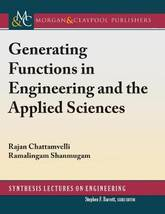This is an introductory book on generating functions (GFs) and their applications.It discusses commonly encountered generating functions in engineering and applied sciences, such as ordinary generating functions (OGF), exponential generating functions (EGF), probability generating functions (PGF), etc. Some new GFs like Pochhammer generating functions for both rising and falling factorials ...
Generating Functions in Engineering and the Applied Sciences
This is an introductory book on generating functions (GFs) and their applications.It discusses commonly encountered generating functions in engineering and applied sciences, such as ordinary generating functions (OGF), exponential generating functions (EGF), probability generating functions (PGF), etc. Some new GFs like Pochhammer generating functions for both rising and falling factorials are introduced in Chapter 2. Two novel GFs called mean deviation generating function (MDGF) and survival function generating function (SFGF), are introduced in Chapter 3. The mean deviation of a variety of discrete distributions are derived using the MDGF. The last chapter discusses a large number of applications in various disciplines including algebra, analysis of algorithms, polymer chemistry, combinatorics, graph theory, number theory, reliability, epidemiology, bio-informatics, genetics, management, economics, and statistics. Some background knowledge on GFs is often assumed for courses in analysis of algorithms, advanced data structures, digital signal processing (DSP), graph theory, etc. These are usually provided by either a course on discrete mathematics or introduction to combinatorics. But, GFs are also used in automata theory, bio-informatics, differential equations, DSP, number theory, physical chemistry, reliability engineering, stochastic processes, and so on. Students of these courses may not have exposure to discrete mathematics or combinatorics. This book is written in such a way that even those who do not have prior knowledge can easily follow through the chapters, and apply the lessons learned in their respective disciplines. The purpose is to give a broad exposure to commonly used techniques of combinatorial mathematics, highlighting applications in a variety of disciplines.
68.200000 USD

## Generating Functions in Engineering and the Applied Sciences

by Ramalingam Shanmugam, Rajan Chattamvelli
Hardback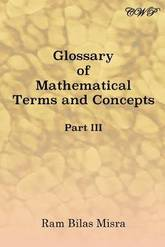Glossary of Mathematical Terms and Concepts (Part III)
178.490000 USD

## Glossary of Mathematical Terms and Concepts (Part III)

by Ram Bilas Misra
Paperback / softback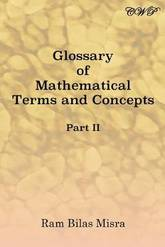Glossary of Mathematical Terms and Concepts (Part II)
178.490000 USD

## Glossary of Mathematical Terms and Concepts (Part II)

by Ram Bilas Misra
Paperback / softback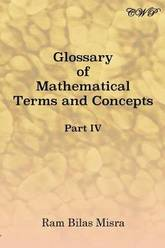Glossary of Mathematical Terms and Concepts (Part IV)
159.590000 USD

## Glossary of Mathematical Terms and Concepts (Part IV)

by Ram Bilas Misra
Paperback / softbackThe book reports a comparative research project about algebra teaching and learning in four countries. Algebra is a central topic of learning across the world, and it is well-known that it represents a hurdle for many students. The book presents analyses built on extensive video-recordings of classrooms documenting the first ...
Encountering Algebra: A Comparative Study of Classrooms in Finland, Norway, Sweden, and the USA
The book reports a comparative research project about algebra teaching and learning in four countries. Algebra is a central topic of learning across the world, and it is well-known that it represents a hurdle for many students. The book presents analyses built on extensive video-recordings of classrooms documenting the first introduction to symbolic algebra (students aged 12 to 14). While the content addressed in all classrooms is variables, expressions and equations, the teaching approaches are diverse. The chapters bring the reader into different algebra classrooms, discussing issues such as mathematization and social norms, the role of mediating tools and designed examples, and teacher beliefs. By comparing classrooms, new insights are generated about how students understand the algebraic content, how teachers instruct, and how both parties deal with difficulties in learning elementary algebra. The book also describes a research methodology using video in search of taken-for-granted aspects of algebra lessons.
125.990000 USD

## Encountering Algebra: A Comparative Study of Classrooms in Finland, Norway, Sweden, and the USA

HardbackImplicative and Disjunctive Prevarieties: Algebraic aspects of Deduction Theorem
210.000000 USD

## Implicative and Disjunctive Prevarieties: Algebraic aspects of Deduction Theorem

by Alexej P Pynko
Paperback / softback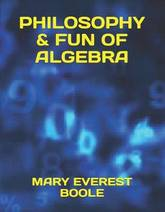Philosophy & Fun of Algebra
181.640000 USD

## Philosophy & Fun of Algebra

by Mary Everest Boole
Paperback / softback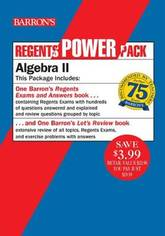Barron's Regents Algebra II Power Pack is the only Algebra II Regents study guide that gives students and teachers comprehensive content review and actual Regents exams as practice. This two-book set provides the key Algebra II content, step-by-step skills review, and real practice that students need to feel prepared for ...
Regents Algebra II Power Pack: Let's Review Algebra II + Barron's Regents Exams and Answers: Algebra II
Barron's Regents Algebra II Power Pack is the only Algebra II Regents study guide that gives students and teachers comprehensive content review and actual Regents exams as practice. This two-book set provides the key Algebra II content, step-by-step skills review, and real practice that students need to feel prepared for the test. Regents Exams and Answers: Algebra II includes: Four actual, administered Regents exams so students can get familiar with the test Comprehensive review questions grouped by topic, to help students refresh skills learned in class Thorough explanations for all answers Score analysis charts to help identify strengths and weaknesses Study tips and test-taking strategies Let's Review: Algebra II includes: Extensive review of all topics on the test including Polynomial Functions, Exponents and Equations, Transformation of Functions, Trigonometric Functions and Graphs, and Using Sine and Cosine Extra exercise problems with answers to help students refresh the skills they learned in class The Power Pack offers a savings of \$3.99 as compared to purchasing the books separately.
20.990000 USD

## Regents Algebra II Power Pack: Let's Review Algebra II + Barron's Regents Exams and Answers: Algebra II

by Gary M. Rubinstein
Paperback / softback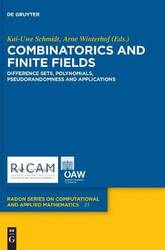The series is devoted to the publication of high-level monographs, surveys and proceedings which cover the whole spectrum of computational and applied mathematics. The books of this series are addressed to both specialists and advanced students. Interested authors may submit book proposals to the Managing Editor or to any member ...
Combinatorics and Finite Fields: Difference Sets, Polynomials, Pseudorandomness and Applications
The series is devoted to the publication of high-level monographs, surveys and proceedings which cover the whole spectrum of computational and applied mathematics. The books of this series are addressed to both specialists and advanced students. Interested authors may submit book proposals to the Managing Editor or to any member of the Editorial Board. Managing Editor Ulrich Langer, Johannes Kepler University Linz, Austria Editorial Board Hansjoerg Albrecher, University of Lausanne, Switzerland Ronald H. W. Hoppe, University of Houston, USA Karl Kunisch, RICAM, Linz, Austria; University of Graz, Austria Harald Niederreiter, RICAM, Linz, Austria Christian Schmeiser, University of Vienna, Austria
146.990000 USD

## Combinatorics and Finite Fields: Difference Sets, Polynomials, Pseudorandomness and Applications

Hardback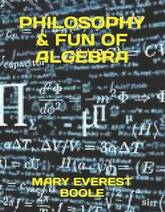Philosophy & Fun of Algebra
179.540000 USD

## Philosophy & Fun of Algebra

by Mary Everest Boole
Paperback / softback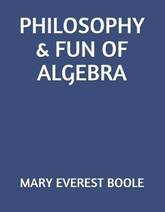Philosophy & Fun of Algebra